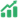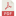# PROFILE OF STUDENTS’ MATHEMATICAL CONNECTION ABILITY IN SOLVING MATHEMATICS PROBLEMS BASED ON VISUALIZER AND VERBALIZER COGNITIVE STYLE

• Nikmatus Savira Aprilianda Universitas Negeri Surabaya
• Susanah Susanah

### Abstract

Mathematical connection ability is the ability of students to connect mathematical ideas and concepts
in a structured way to solve various problems both inside and outside mathematics. Mathematical connection
ability plays an important role in the process of solving mathematical problems. Cognitive style is one of the
factors that effect mathematical connection abilities. This research is a qualitative descriptive study that aims to
describe the students' mathematical connection skills with visualizer and verbalizer cognitive styles in solving
mathematics problems. The research subjects consisted of two grade IX students who each had visualizer and
verbalizer cognitive styles. The instrument used was the researcher herself, VVQ (visualizer verbalizer
questionnaire), a mathematical connection ability test, and interview guidelines. In this study, the material of
plane area and Pythagorean theorem are used for test mathematical connection skills. The results obtained are
students with visualizer cognitive style get a good category for their mathematical connection ability in solving
mathematics problems because they meet seven good indicators and one sufficient indicator from the
mathematical connection ability in solving problems indicator, while students with verbalizer cognitive style get
sufficient categories because they meet three good indicators, four sufficient indicators, and one less indicator
from mathematical connection ability in solving problems indicator. Therefore, teachers are expected to be able
to train students with questions in the context of everyday life that have a higher level of mathematical
connection so that they can improve their connection skills and also train with variety exercices presentation so
student with each cognitive style can be trained to understand a given problem.

Published
2022-03-21
Section
ArticlesAbstract Views: 84PDF Downloads: 108